Prime Factors Operation Without Filter ConditionWhen no filter condition is set the program examines all numbers in the specified range from the start number to the end number. For example, the input shown at right produces (by clicking on the Factor, display, count or plot button) the following output (in about a second):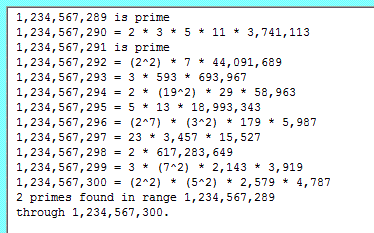When the Show number of prime factors option is selected (and the Factor button is clicked on) we get: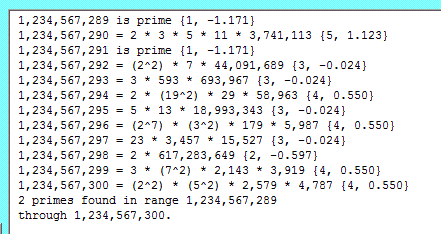Of the two numbers in parentheses, e.g. (5, 1.123) in the 2nd line, the first number is the number of prime factors (in this example there are five: 2, 3, 5, 11 and 3,741,113). The second number is the EK value (defined here).

When the Display primes only option is selected we obtain: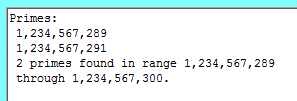Requesting prime pairs only on the same range of numbers produces the following output: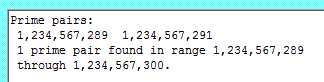The largest prime pair which can be discovered using this program is 2,147,482,949 and 2,147,482,951.  It is not known whether there are an infinite number of prime pairs, but this is conjectured to be so.

Prime Factors allows you to find all proper factors of a number (i.e., all factors other than 1 and the number itself). E.g.:You can get all proper factors of a range of numbers, e.g.: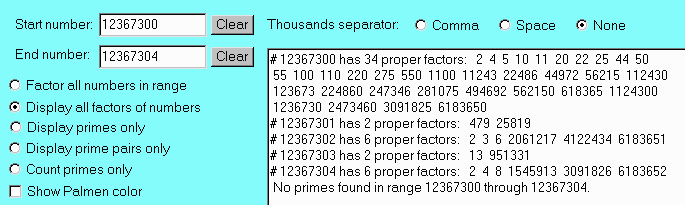A lengthy output can be copied to the clipboard and then pasted into a word processor such as Notepad or Wordpad (from which it can be printed or saved to disk). The text in the output window is editable, so you can delete parts of it or add comments as needed. The state of the program can be saved at any time, allowing you to explore another line of thought and then return to the saved state by restoring it. The program saves its state when you quit and restores it when it starts up again.

As regards speed, here are some timing results (all from runs on a 600 MHz Pentium III PC):

• The number of primes in the range 1,000,000 through 2,000,000 (namely, 70,435) was calculated in 26 seconds.
• The 1,409 primes in the range 2,000,000,000 through 2,000,030,000 were calculated and displayed in 37 seconds.
• The prime decompositions of all 501 numbers in the range 1,000,000 through 1,000,500 (with 39 primes found) were calculated and displayed in 23 seconds.
• The proper factors of the 101 numbers in the range 2,000,000,000 through 2,000,000,100 were calculated and displayed in 31 seconds (with 6 primes found).
• The 862 proper factors of 1,914,412,500, which has prime decomposition 22.32.55.7.11.13.17, were calculated and displayed in ascending order in a few seconds.

If a calculation goes on for too long then it can be stopped by clicking on a Stop button: